﻿ 证明D-Finite类数学猜想的新方法 A New Method to Prove Mathematical Conjecture of D-Finite Class

Pure Mathematics
Vol. 09  No. 03 ( 2019 ), Article ID: 30296 , 7 pages
10.12677/PM.2019.93046

A New Method to Prove Mathematical Conjecture of D-Finite Class

—Talking about the Differential Theorem of Morley Triangle

Jun Yang1, Zhicheng Guo2*

1Beijing Institute of Technology, Zhuhai, Zhuhai Guangdong

2Northern Design and Research Institute, Shijiazhuang HebeiReceived: Apr. 25th, 2019; accepted: May 5th, 2019; published: May 20th, 2019ABSTRACT

This paper presents and proves the Morley differential theorem (Gauss curvature pointer fineness theorem) based on Morley’s theorem. According to the conclusion of the three-point collinearity of this theorem, a correlation model between the right triangle and its mirror image and the Möbius function is constructed, which lays a foundation for the further study of the interpolation algorithm and discrete properties of the Möbius function. This theorem reveals that the nature of functional is functional “one-dimensional”, which lays a foundation for further extending the geometric properties of functional one-dimensional to functional “plane”. The theorems, corollaries and open problems in this paper reveal the properties of limit cycles of vector fields of first order polynomials and the research approaches of vector fields of quadratic polynomials. The new method to prove non-commutative D-finite class conjectures is given.

Keywords:Morley’s Triangles, Gaussian Curvature, D-Finite, Conformal Mappings

——从Morley三角形的微分定理谈起

1北京理工大学珠海学院，广东 珠海

2北方设计研究院，河北 石家庄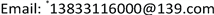1. 引言

Galois群论告诉我们：采用几何作图(有限次)的方法不能画出一个任意角的三等分线。然而有限次循环采用几何作图的方法依次画出三角形每一个内角的两等分线并逐渐逼近画出三等分线，可以得到著名的Morley三分线定理  ：一个三角形的三个内角的三等分线共六条线，与三角形三条边最近的两条三等分线分别相交于三点，将三点两两连线就构成了等边三角形。如图1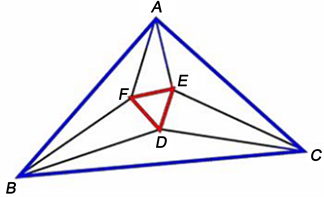Figure 1. Morley’s equilateral triangle

2. Gauss曲率及其指针的概念

$K=\underset{F\to 0}{\mathrm{lim}}\frac{G}{F}$

3. Morley三分线微分定理研究

$\begin{array}{l}\angle AEF=\angle BDF={60}^{\circ }+\frac{1}{3}\angle C,\\ \angle BFD=\angle CED={60}^{\circ }+\frac{1}{3}\angle A,\\ \angle CDE=\angle AFE+\frac{1}{3}\angle B={60}^{\circ }+\frac{1}{3}\angle B\end{array}$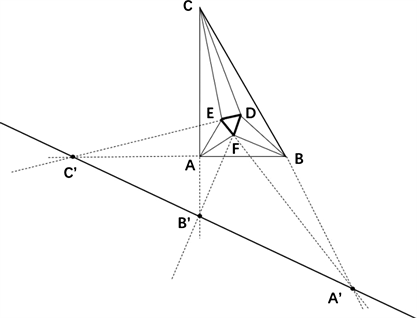Figure 2. Morley’s triangle and opposite intersection ${A}^{\prime },{B}^{\prime },{C}^{\prime }$

$\begin{array}{l}\angle D{C}^{\prime }A=\frac{1}{3}\left(\angle C-\angle B\right),\\ \angle E{A}^{\prime }B=\frac{1}{3}\left(\angle A-\angle C\right),\\ \angle F{B}^{\prime }C=\frac{1}{3}\left(\angle B-\angle A\right)\end{array}$

${A}^{\prime },{B}^{\prime },{C}^{\prime }$ 做圆，存在唯一的圆弧和圆心。当 $2 为有理数时，n内等分三角形的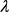大于0，它与三个角度 $\angle D{C}^{\prime }A,\angle E{A}^{\prime }B,\angle F{B}^{\prime }C$ 的符号乘积相同，视为圆弧和圆心同向(凹多边形)；当 $3 为有理数时，n内等分三角形的 $\lambda$ 小于0，它与三个角度 $\angle D{C}^{\prime }A,\angle E{A}^{\prime }B,\angle F{B}^{\prime }C$ 的符号乘积相反，视为圆弧和圆心反向(凸多边形)。显然，当 $n=3$ 时，圆心只能在圆弧上。

$n\to \infty$ 时，n等分三角形∆ABC内角的靠近三边的三个交点 ${A}_{1},{B}_{1},{C}_{1}$ 位于三角形的三边上，且三角形∆A1B1C1的边长的长短顺序与三角形∆ABC的边长的长短顺序相反。如果继续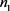等分新三角形∆A1B1C1的内角，当 ${n}_{1}\to \infty$ 时，重复上述过程就得到与三角形∆ABC的边长的长短顺序相同的第二个新三角形∆A2B2C2。如果把这个过程重复无穷多次(即 ${n}_{\to \infty }\to \infty$ )，则在三角形∆ABC的内部得到了一个定点，记为 。

4. Gauss曲率指针及其符号的细数研究

Gauss曲率指针符号的定义：三等分的内等分三角形是二元函数 $\lambda$ 的唯一改变符号的点，而 $\lambda$ 的变化与Gauss曲率的指针方向相关，因此称 $\lambda$ 为Gauss曲率的指针符号。

Gauss指针的进一步细分属于非交换D-finite生成函数的研究范畴，本文揭示了这种生成函数的本质。

$2 时，曲面上某一点k始终在三角形∆ABC中，k点的曲率可以由三角形∆ABC收缩为一点得到。显然，如果直角三角形∆ABC中的线段 $|AB|<|BC|$ ，那么共点直线上的线段长度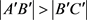。我们用比值的乘积 $\frac{|AB|}{|BC|}\cdot \frac{|{A}^{\prime }{B}^{\prime }|}{|{B}^{\prime }{C}^{\prime }|}$ 或者比值的商 $\frac{|AB|}{|BC|}\cdot \frac{|{B}^{\prime }{C}^{\prime }|}{|{A}^{\prime }{B}^{\prime }|}$ 表示Gauss曲率指针的精细结构(或细数Fine numbers)。人们通常也把点k的Gauss曲率指针记为Möbius函数 $\mu \left(k\right)=+1$ 。同理，把三角形∆ABC的镜像 $\Delta \stackrel{¯}{ABC}$ 收缩为一点得到的Gauss曲率指针记为Möbius函数 $\mu \left(\stackrel{¯}{k}\right)=-1$

$AF=\frac{\mathrm{sin}\beta }{\mathrm{sin}\left(\alpha +\beta \right)}\cdot AB=\frac{2r\cdot \mathrm{sin}\beta \cdot \mathrm{sin}3\gamma }{\mathrm{sin}\left(\frac{\text{π}}{3}-\gamma \right)}=8r\cdot \mathrm{sin}\beta \cdot \mathrm{sin}\gamma \cdot \mathrm{sin}\left(\frac{\text{π}}{3}+\gamma \right)$

$AE=8r\cdot \mathrm{sin}\beta \cdot \mathrm{sin}\gamma \cdot \mathrm{sin}\left(\frac{\text{π}}{3}+\beta \right)$

$E{F}^{2}=A{E}^{2}+A{F}^{2}-2AE\cdot AF\cdot \mathrm{cos}\alpha$

$EF=8r\mathrm{sin}\left(\alpha +\theta \right)\cdot \mathrm{sin}\left(\beta +\varphi \right)\cdot \mathrm{sin}\left(\gamma +\psi \right)$

5. 开放性问题的现状和展望

Hilbert第16个问题在Hilbert提出的23个问题中进展最小。Hilbert第16个问题是正则变分问题的解是否一定解析——即任意向量场的正则图是否属于分析性质，其中的一部分是求多项式(即平面)向量场的极限环数的上界，极限环是指向量场的孤立周期轨道。

A New Method to Prove Mathematical Conjecture of D-Finite Class[J]. 理论数学, 2019, 09(03): 344-350. https://doi.org/10.12677/PM.2019.93046

1. 1. Morley, F. (1903) Orthocentric Properties of the Plane n-Line. Transactions of the American Mathematical Society, 4, 1-12.
https://doi.org/10.2307/1986445

2. 2. Braude, E.J. (2016) Generalizing the Morley Trisector and Various Theorems with Realizability Computations. arXiv:1603.03463 [cs.CG]

3. 3. Karamzadeh, O.A.S. (2014) Is John Conway’s Proof of Morley’s Theorem the Sim-plest and Free of a Deus ex Machina? The Mathematical Intelligencer, 36, 4-7.
https://doi.org/10.1007/s00283-014-9481-1

4. 4. Letac, A. (1939) Solution (Morley’s Triangle), Problem No. 490. Sphinx, 9, 46.

5. 5. Dobbs, W.J. (1938) Morley’s Triangle. Mathematical Gazette, 22, 50-57, and 189 for comment.
https://doi.org/10.2307/3607446

6. 6. Bremner, A., Goggins, J.R., Guy, M.J.T. and Guy, R.K. (2000) On Rational Morley Triangles. Acta Arithmetica, 2, 177-187.
https://doi.org/10.4064/aa-93-2-177-187

7. 7. Stanley, R P. (2015) Catalan Number. Cambridge University Press, Cambridge.

8. 8. Dulac, H. (1923) Sur les Cycles Limites. Bulletin de la Société Mathématique de France, 51, 45-188.
https://doi.org/10.24033/bsmf.1031

9. 9. Chicone, C. and Shafer, D.S. (1983) Separatrix and Limit Cycles of Quadratic Systems and Dulac’s Theorem. Transactions of the American Mathematical Society, 278, 585-612.
https://doi.org/10.1090/s0002-9947-1983-0701513-x

10. NOTES

1注：限制性条件相当于歌德尔的固定符号，等价意味着具有相同的形式演算系统“PM”。

*通讯作者。